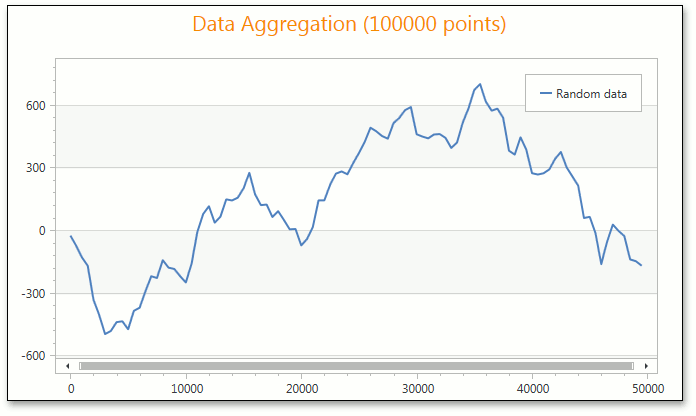21.2 (EAP/Beta)
21.1
20.2
20.1
19.2
19.1
18.2
18.1
17.2

# ManualNumericScaleOptions.AggregateFunction Property

Gets or sets the function that is used to aggregate numeric axis data when one of the AxisX2D.NumericScaleOptions, AxisX3D.NumericScaleOptions, or CircularAxisX2D.NumericScaleOptions properties is set to ManualNumericScaleOptions.

Namespace: DevExpress.Xpf.Charts

Assembly: DevExpress.Xpf.Charts.v21.1.dll

## Declaration

``public AggregateFunction AggregateFunction { get; set; }``

## Property Value

Type Description
AggregateFunction

An AggregateFunction enumeration value.

Available values:

Name Description
None

The aggregate function is not applied.

Average

Aggregates data by calculating the average value for a selected numeric or date-time interval.

Minimum

Aggregates data by calculating the minimum value for a selected numeric or date-time interval.

Maximum

Aggregates data by calculating the maximum value for a selected numeric or date-time interval.

Sum

Aggregates data by calculating the summary for a selected numeric or date-time interval.

Count

Aggregates data by calculating the number of non-null values for a selected numeric or date-time interval.

Histogram

Aggregates data by calculating the number of all points for a selected numeric or date-time interval.

Financial

Aggregates financial data for a selected interval into a single high-low-open-close data point, so that the high value equals the highest value of all data points in this interval; the low value equals the lowest value of all data points; the open value equals the value of the first data point and the close value equals the value of the last data point.

Custom

Aggregates data using the custom CustomAggregateFunction calculator.

## Remarks

The image below shows numeric data aggregation in the Manual scale mode using the default Average function.Note

The AggregateFunction property is set to AggregateFunction.Average by default.

To disable numeric data aggregation, set the AggregateFunction property to AggregateFunction.None.

## Example

This example demonstrates how to use the manual numeric scale options of the X-axis.

When the AxisX2D.NumericScaleOptions property is set to ManualNumericScaleOptions, you can manually define the ManualNumericScaleOptions.GridAlignment, ManualNumericScaleOptions.MeasureUnit and ManualNumericScaleOptions.AggregateFunction properties.

To use the automatic numeric scale options, set the AxisX2D.NumericScaleOptions property to AutomaticNumericScaleOptions and choose the appropriate AutomaticNumericScaleOptions.AggregateFunction.

To prevent the axis scale from being divided into intervals (and thus prevent chart data from being aggregated), set the ManualNumericScaleOptions.AggregateFunction or AutomaticNumericScaleOptions.AggregateFunction property to None.

``````<Window
xmlns="http://schemas.microsoft.com/winfx/2006/xaml/presentation"
xmlns:x="http://schemas.microsoft.com/winfx/2006/xaml"
xmlns:dxc="http://schemas.devexpress.com/winfx/2008/xaml/charts"
x:Class="AggregationNumericData.MainWindow"
Title="MainWindow" Height="350" Width="525">
<Grid>
<dxc:ChartControl >
<dxc:ChartControl.Legend>
<dxc:Legend/>
</dxc:ChartControl.Legend>
<dxc:XYDiagram2D>
<dxc:XYDiagram2D.AxisX>
<dxc:AxisX2D GridLinesVisible="True">
<dxc:AxisX2D.NumericScaleOptions>
<dxc:ManualNumericScaleOptions MeasureUnit="100" GridAlignment="1000"
AggregateFunction="Maximum" GridSpacing="2"/>
</dxc:AxisX2D.NumericScaleOptions>
</dxc:AxisX2D>
</dxc:XYDiagram2D.AxisX>
<dxc:LineSeries2D DataSource="{Binding Path=Series}"
ArgumentDataMember="Argument" ValueDataMember="Value"/>
</dxc:XYDiagram2D>
</dxc:ChartControl>
</Grid>
</Window>
``````